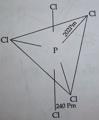State reasons for each of the following :

(i) All the P-Cl bonds in $PC{l}_{5}$ molecule are not equivalent.

(ii) Sulphur has greater tendency for catenation than oxygen.

Concept Videos :-

#2 | 15th Group: Properties: II
#3 | 15th Group: Ammonia

Concept Questions :-

group 15 ,preparation and properties

(i)The $PC{l}_{5}$ molecule has $s{p}^{3}d$ hybridization and trigonal bipyramidal geometry. Therefore it has 3 equatorial P-Cl bonds and two axial P-Cl bonds. Since two axial P-Cl bonds are repelled by 3 bond pairs while 3 equatorial bonds are repelled by two bond pairs, so axial bonds are longer than equatorial bonds.(ii) The greater catenation tendency of sulphur is due to two reasons :

(a) The lone pair of electrons feels more repulsion in O-O bond than S-S bond due to its small size and thus S-S forms strong bond.

(b) As the size of atom increases down the group from O-PO, the strength of bond increases and therefore catenation tendency also increases.

Difficulty Level:

• 82%
• 10%